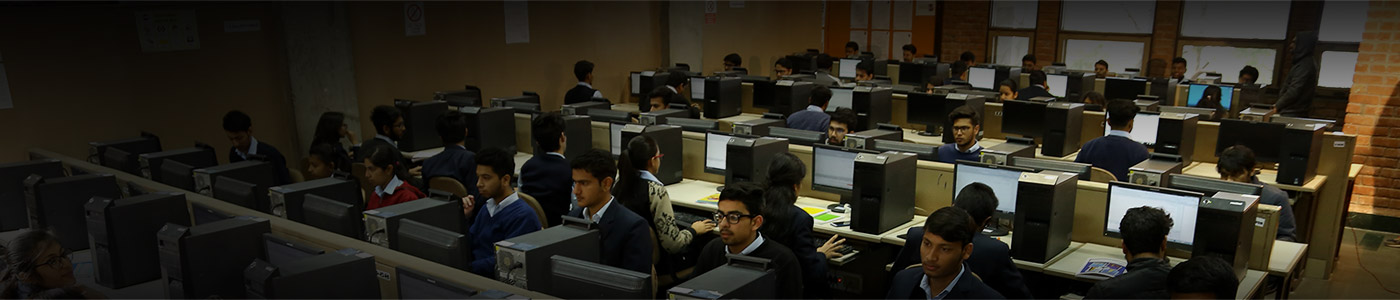# Algorithms and Parallel Computing

The Algorithms and Parallel computing group at the Jaypee University of Information Technology (JUIT) is part of the JUIT’s Computer Science and Information Technology Department. The primary goal of the Algorithms and Parallel Computing group is to provide a mathematical design and engineering of computer algorithms, and to use these algorithms to produce better applications, protocols, and systems. Researchers in our group explore a variety of algorithm types and areas of applications. Some of the different research domains of our group is as follows:

• Combinatorial algorithms
• Randomized algorithms
• Parallel and Distributed Algorithms
• Distributed Synchronization
• Self-stabilizing Algorithms
• Nature Based Algorithm
• Theory of Computation
• Programming languages.